# Find the date difference between rows

Data Pipeline

When wanting to calculate the difference in time between two date columns, the Date difference formula option in the Add Column or Edit Column Pipeline steps can be utilized.

In the event where you only have one date column but would like to calculate the difference between each row, you can instead use the datediff() function in the Data Pipeline.

## Using the lag() function

An easy way to do this is to use the lag() preset function in your Add Column or Edit Column Pipeline steps to create a start column to use as part of your date calculation.

In the example below, we’ll be looking to calculate the number of days between user login times.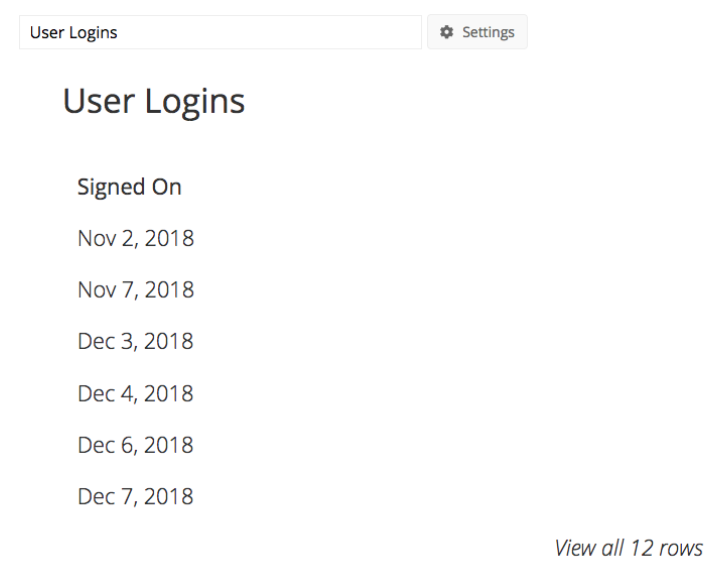1. First, query the column containing the login dates and run the query. For this example, we’ll use our Signed On column.

2. Add a new column in the Data Pipeline. This column will act as the start date to calculate the date difference. Here we’ll name the column “Signed On 2”. We’ll then want to set the Formula Type as Lag and set a Row Offset of 1. Click Apply & Close when done.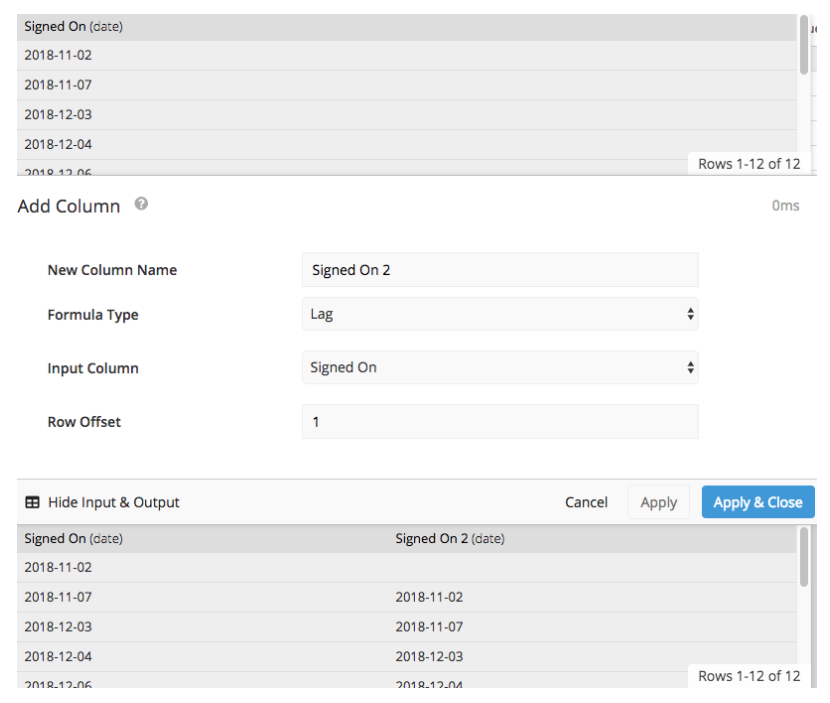3. After creating the offset column, we’ll want to create another column to calculate the number of days between login dates. To do this, we’ll add a column and select Date difference from the Formula Type dropdown menu. Then, select the start and end columns as well as the time units. Click Apply & Close when done.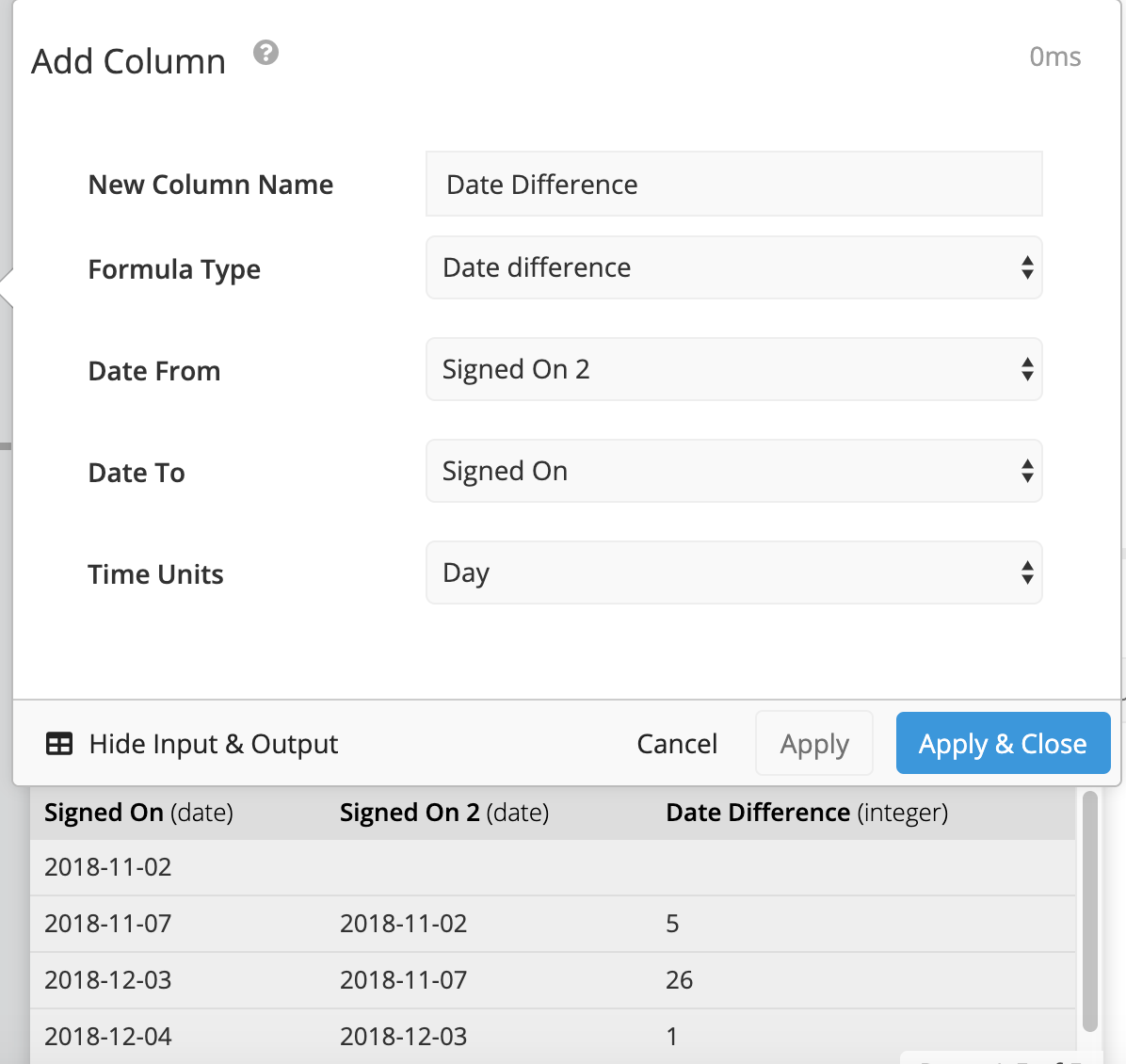Instead of the preset option, you can also use the datediff() formula below as part of a custom formula, replacing the Start Date Column and End Date Column with the names of your columns and unit with the time unit you’d like to calculate:

``````datediff("Start Date Column","End Date Column",'unit'(optional))
``````

Note: If you choose not to specify a unit in the formula, it will default to calculate days.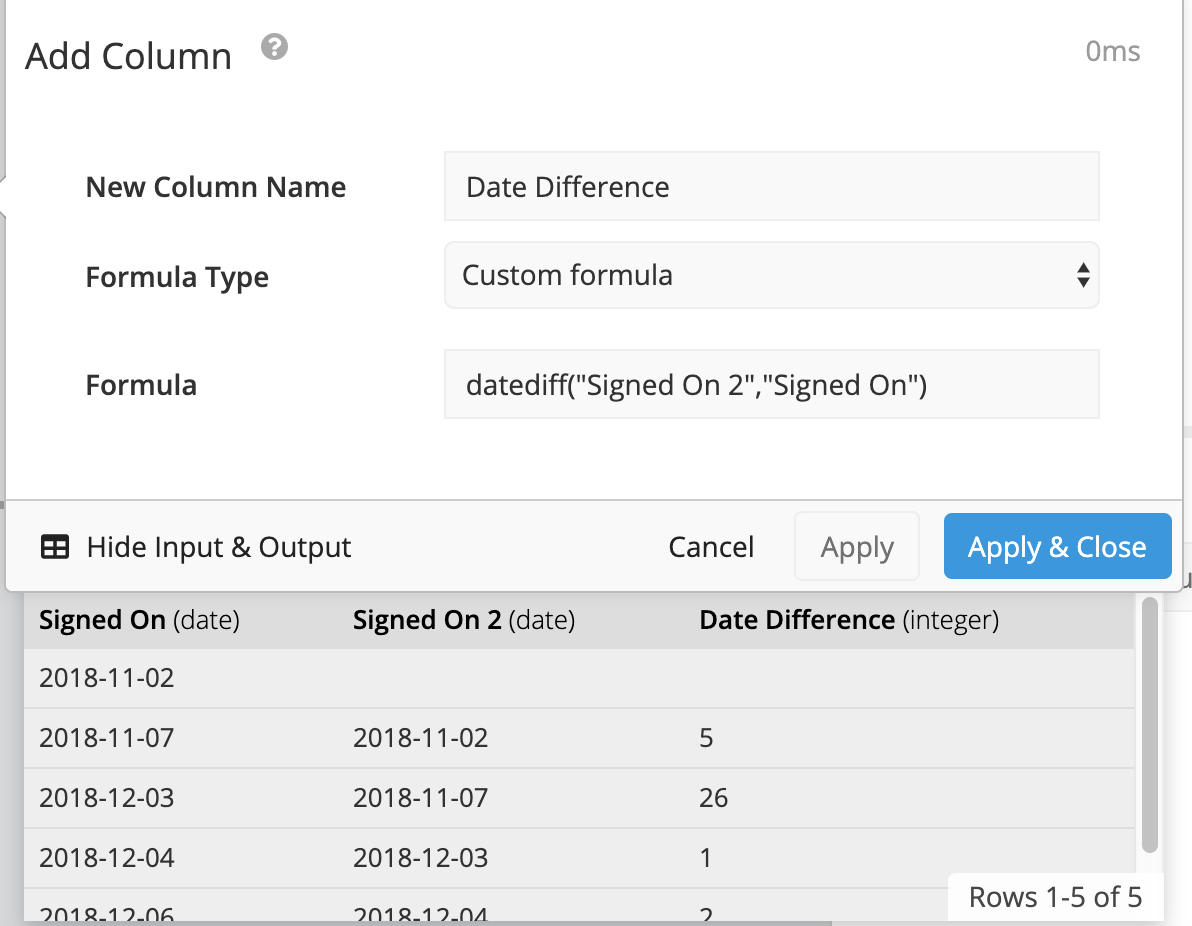Another option is to skip adding an additional lag column and use the lead() Window Function in place of the end column in your datediff() formula. This function acts similarly to the lag() function we used above and will populate with the end date automatically.

To do this, add a column in the Data Pipeline and choose Custom formula from the Formula Type dropdown. Then, add the formula below to the Formula field, replacing Date Column in the formula below with the name of your date column:

``````datediff("Date Column",(lead("Date Column", 1) over (order by "Date Column"(optional))))
``````

Here, we simply wanted to keep the current order of date rows, therefore, we omitted the order by clause from the formula as follows:

``````datediff("Signed On",(lead("Signed On", 1) over ()))
``````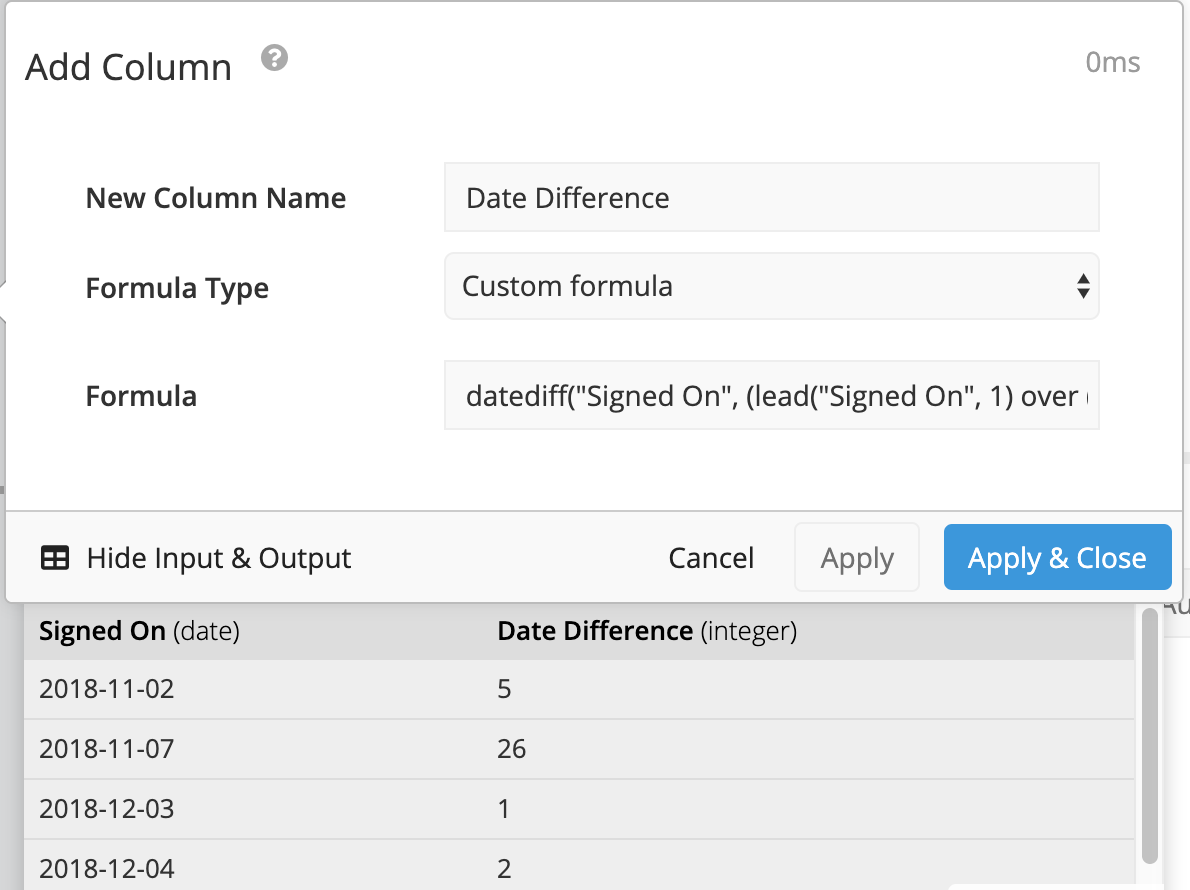You now have the number of days between login dates and can perform additional calculations as needed.